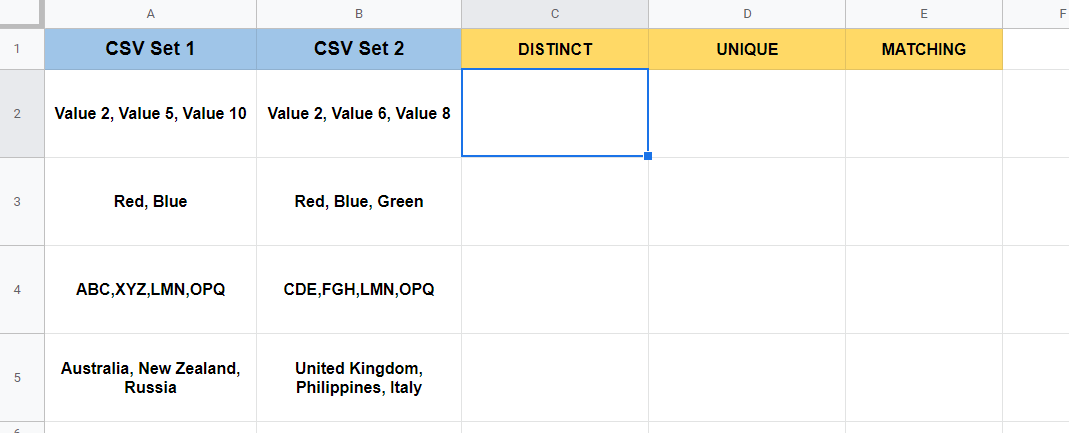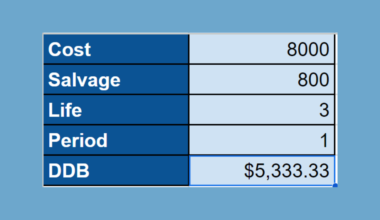# How to Compare Comma-Separated Values in Google Sheets

This article will show you different ways we can use Google Sheets formulas to compare cells with comma-separated values.

Comma-separated values or CSVs are a common way data is formatted. Instead of each value taking up one cell, each value is found in a single string, separated by commas.

This guide will show you three ways we can compare CSVs in Google Sheets.

First, we’ll look at distinct values. These are values that do not have duplicates. They are either unique to the first or second set.

Second, we’ll take a look at two CSV sets and find unique values from both. This means that we’ll combine all elements from both sets and remove any duplicates.

Third, we’ll look at matching values. These are values that can be found in both sets.

Let’s take a look at a few examples of some possible use cases for these formulas.

We have a spreadsheet with two CSV sets. The first is a list of clients with pending orders. The second is a list of clients who have already paid you upfront. Using our distinct formula, we can find out all the sellers from both lists.

We can also use the matching formula to see clients with pending orders that have already been paid upfront. This can help you prioritize which clients to prioritize during shipping.

As another example, we can also consider a spreadsheet that contains two comma-separated strings of movie suggestions for an upcoming event. We can use the unique formula to generate a master list of suggested movies from both lists.

These use cases are just one situation where we might have to compare CSVs in Google Sheets. In the next section, we’ll look at concrete examples of these formulas in use and explain how they work.

Let’s learn how to write these formulas ourselves in Google Sheets.

## A Real Example of Comparing Comma-Separated Values in Google Sheets

Let’s look at a real example of comparing comma-separated strings in a Google Sheets spreadsheet.

In the sheet below, we’ve created a table that uses Google Sheets formulas to compare two comma-separated strings. For example, we have colors as our elements in the second row.

The distinct formula indicates that only Green belongs to just one set. The matching formula tells us that both Red and Blue appear in both sets. Finally, the unique formula lists off all the values found in both lists.To get the distinct values, we just need to use the following formula:

`=TEXTJOIN(",",true,unique(ArrayFormula(trim(split(textjoin(", ", true,A2:B2),","))),true,true))`

This formula mainly uses the `SPLIT` and `UNIQUE` function to get the text from both CSV sets and get all the distinct values. The `TRIM` function allows us to remove any whitespace which might appear before or after each comma.

To get the unique values, we just need to use the following formula:

`=TEXTJOIN(", ",true,unique(ArrayFormula(trim(split(textjoin(", ", true,A2:B2),","))),true,false))`

This formula also uses `SPLIT` and `UNIQUE` to get distinct values. The main difference here is that values that appear more than once remain in the output.

To get the matching values, we just need to use the following formula:

`=TEXTJOIN(", ",TRUE,filter(trim(split(A2,",")),regexmatch(trim(split(A2,",")),textjoin("|",true,trim(split(B2,","))))))`

This formula uses the `REGEXMATCH` and `FILTER` functions to find matches between the first and second sets. We use `REGEXMATCH` since we want to match values in the second set that appears in the first set.

If you’re ready to try out these functions in Google Sheets, let’s begin writing it ourselves!

## How to Compare Comma-Separated Values in Google Sheets

In this section, we will go through each step needed to start comparing comma-separated strings in Google Sheets. This guide will show you how to set up the spreadsheet example seen earlier.

Follow these steps to create a similar worksheet:

1. First, select the cell that will include our formula. In this example, we’ll fill out the column for distinct elements.2. Next, we simply type the equal sign ‘=‘ to begin the function, followed by our distinct formula.3. You can now use your mouse to drag the formula down and fill out the rest of the column.4. Lastly, you can proceed to do the same steps to fill out the unique and matching elements.That’s all you need to remember on how to compare CSVs in Google Sheets. This step-by-step guide shows how easy it is to find distinct, unique, and matching values in cells formatted like a CSV.

CSV data is a common format, and it’s helpful to know what to do when you come across this type of data. You may also look into the `IMPORTDATA` function if you need to import a CSV file hosted on the web.

You can now use these formulas in Google Sheets together with the various other Google Sheets formulas available to create great worksheets that solve your use case.

Are you interested in learning more about what Google Sheets can do? Stay notified of new guides like this by subscribing to our newsletter!Our goal this year is to create lots of rich, bite-sized tutorials for Google Sheets users like you. If you liked this one, you'd love what we are working on! Readers receive ✨ early access ✨ to new content.

##### You May Also Like## How to Use DDB Function in Google Sheets

This guide will explain how to use the DDB function in Google Sheets. When we need to calculate…## How to Link Data from a Different Tab in Google Sheets

When working with Google Sheets, it might be helpful to link data from a different tab in your…## How to Use FILTER Function in Google Sheets

The FILTER function in Google Sheets is useful to filter and return the rows or columns in a…## How to Use the DISC Function in Google Sheets

The DISC function in Google Sheets is used to calculate the discount rate of a security, based on the…